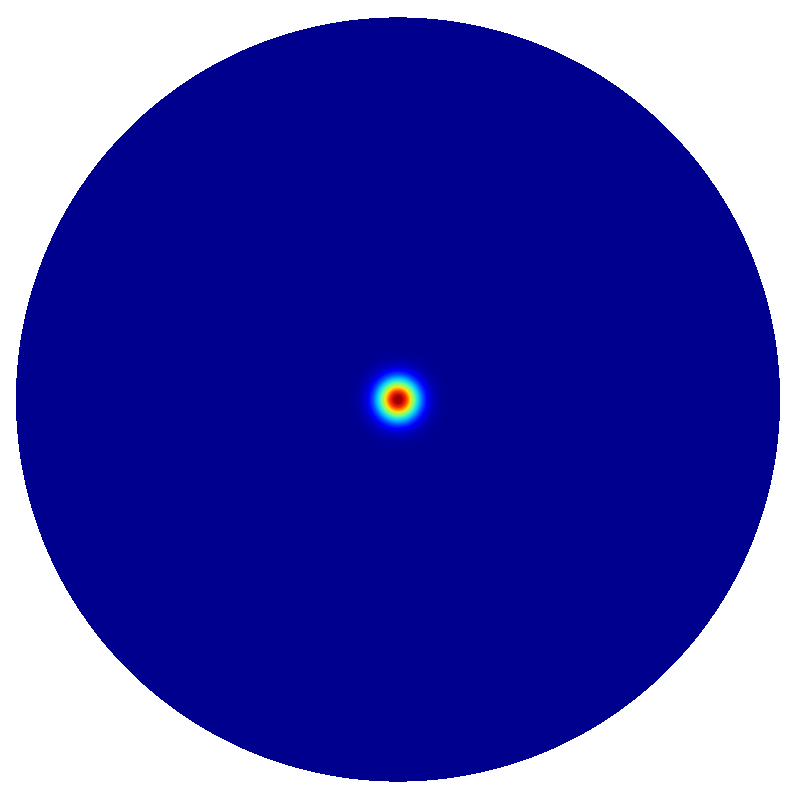# Far Field¶

This example demonstrates computation of the far field corresponding to resonance modes of a VCSEL cavity. The physical design of the VCSEL cavity is identical to the example in the parent folder VCSEL.

The far field is computed with the post-process `FarField` placed in the `project.jcmp` file:

```PostProcess {
FarField {
FieldBagPath = "./project_results/fieldbag.jcm"
OutputFileName = "./project_results/farField.jcm"
Rotation = X:Z:-Y
}
}
```

The `Rotation` definition is needed to match the 3D convention of the polar coordinate system, where the-axis is the optical axis (in contrast to the 2D computational domain convention whereis the axis perpendicular to the cross-section computational domain). You can either load the far field input Matlab or use `JCMsuite` which yields the following polar far field plots: